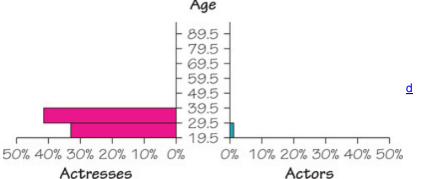×
Get Full Access to Elementary Statistics - 12 Edition - Chapter 2.4 - Problem 19bsc
Get Full Access to Elementary Statistics - 12 Edition - Chapter 2.4 - Problem 19bsc

×

# Frequency Polygon. In Exercises, construct the frequencyISBN: 9780321836960 18

## Solution for problem 19BSC Chapter 2.4

Elementary Statistics | 12th Edition

• Textbook Solutions
• 2901 Step-by-step solutions solved by professors and subject experts
• Get 24/7 help from StudySoup virtual teaching assistantsElementary Statistics | 12th Edition

4 5 1 292 Reviews
29
5
Problem 19BSC

Back­-to­-Back Relative Frequency Histograms When using histograms to compare two data sets, it is sometimes difficult to make comparisons by looking back and forth between the two histograms. A back­to­back relative frequency histogram has a format that makes the comparison much easier. Instead of frequencies, we should use relative frequencies (percentages or proportions) so that the comparisons are not difficult when there are different sample sizes. Use the relative frequency distributions of the ages of Oscar­-winning actresses and actors from Exercise 15 in Section 2­2, and complete the back­to­back relative frequency histograms shown   below. Then use the result to compare the two data sets.Step-by-Step Solution:
Step 1 of 3

Solution 19BSC

The magnitudes appear approximately to be normal. The frequency start 5, and then increase to frequency 15, then increase to 19, then decrease to a frequency 7 and then decrease to two.

Step 2 of 3

Step 3 of 3

##### ISBN: 9780321836960

Elementary Statistics was written by and is associated to the ISBN: 9780321836960. This textbook survival guide was created for the textbook: Elementary Statistics, edition: 12. Since the solution to 19BSC from 2.4 chapter was answered, more than 438 students have viewed the full step-by-step answer. The answer to “?Back­-to­-Back Relative Frequency Histograms When using histograms to compare two data sets, it is sometimes difficult to make comparisons by looking back and forth between the two histograms. A back­to­back relative frequency histogram has a format that makes the comparison much easier. Instead of frequencies, we should use relative frequencies (percentages or proportions) so that the comparisons are not difficult when there are different sample sizes. Use the relative frequency distributions of the ages of Oscar­-winning actresses and actors from Exercise 15 in Section 2­2, and complete the back­to­back relative frequency histograms shown below. Then use the result to compare the two data sets.” is broken down into a number of easy to follow steps, and 104 words. The full step-by-step solution to problem: 19BSC from chapter: 2.4 was answered by , our top Statistics solution expert on 03/15/17, 10:30PM. This full solution covers the following key subjects: frequency, oil, polygon, million, barrels. This expansive textbook survival guide covers 121 chapters, and 3629 solutions.

Unlock Textbook Solution